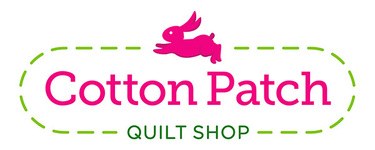table div table+table div table{width:100%;padding:0}table div table+table div table img{width:96.23%;padding:0;float:none}table div table+table div table td{width:100%;padding:0 1.88% 18px}/* styles */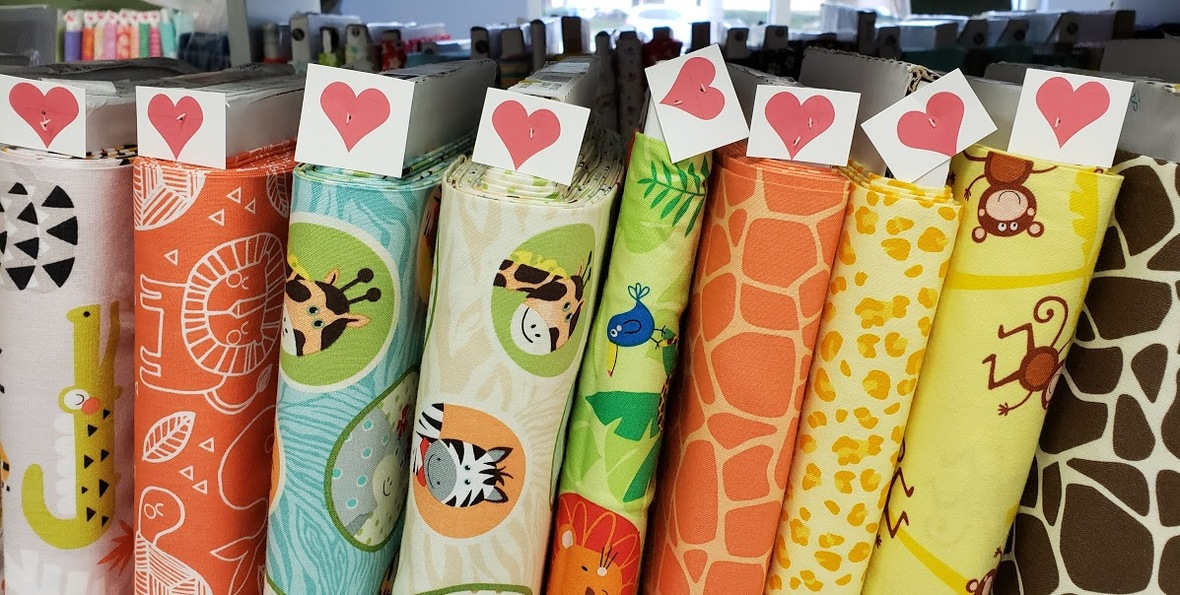# Sweetheart Sale!

Find the bolts tagged with a red heart and save 25% - that makes it LESS than \$10 a yard! (1/2 yard minimum)

## But wait...there's more...

All Markdown fabric is an ADDITIONAL 25% OFF! - that makes it LESS than \$7 a yard! (1 yd minimum)

Sale is Thursday, February 14 through Sunday, February 17
Shop early for best selection!

 table div table+table+table+table+table div table{width:100%;padding:0}table div table+table+table+table+table div table img{width:96.23%;padding:0;float:none}table div table+table+table+table+table div table td{width:100%;padding:0 1.88% 18px}/* styles */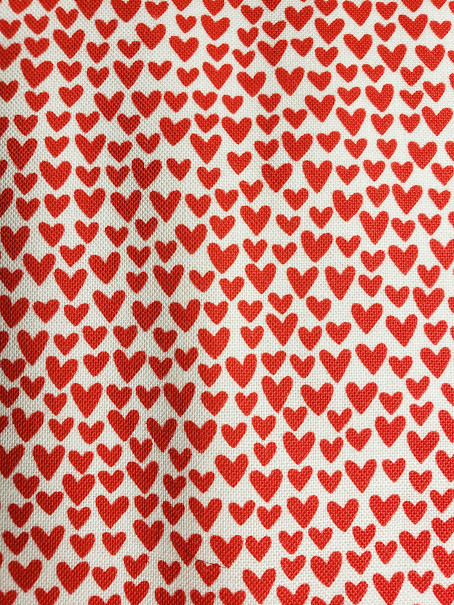# Psst, we (heart) our customers!

Shop on Valentine's Day and receive a FREE hearts fat quarter, just cuz we love you shopping with us!# Psst, we (heart) our customers!

Shop on Valentine's Day and receive a FREE hearts fat quarter, just cuz we love you shopping with us!

 table div table+table+table+table+table+table+table+table div table{width:100%;padding:0}table div table+table+table+table+table+table+table+table div table img{width:96.23%;padding:0;float:none}table div table+table+table+table+table+table+table+table div table td{width:100%;padding:0 1.88% 18px}/* styles */table div table+table+table+table+table+table+table+table+table div table{width:100%;padding:0}table div table+table+table+table+table+table+table+table+table div table img{width:96.23%;padding:0;float:none}table div table+table+table+table+table+table+table+table+table div table td{width:100%;padding:0 1.88% 18px}/* styles */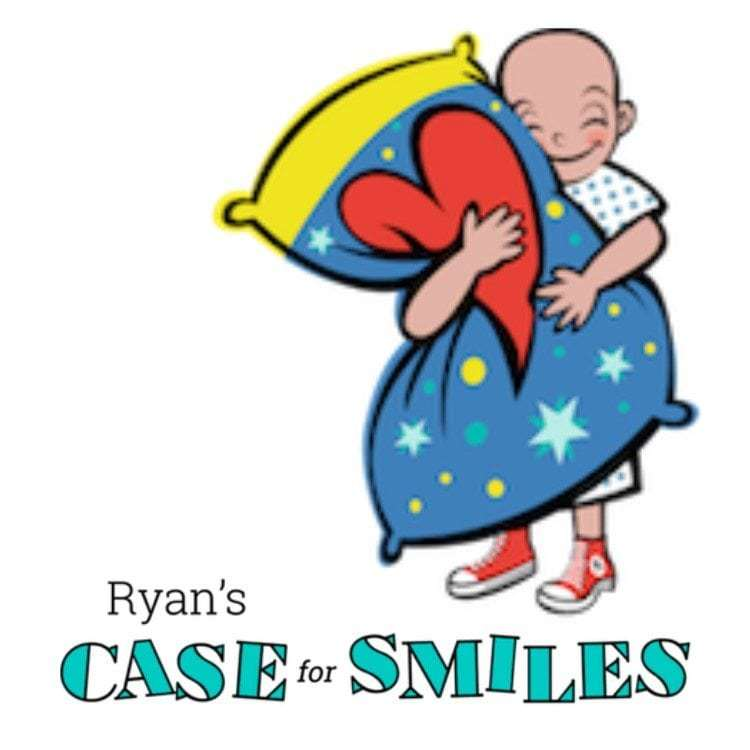# Pillowcase Sew Day!

## Thursday, February 14

10am to 2pm

A big "THANK YOU" to Judy B for her generous contribution of zip loc bags and color catchers!

 table div table+table+table+table+table+table+table+table+table+table+table+table div table{width:100%;padding:0}table div table+table+table+table+table+table+table+table+table+table+table+table div table img{width:96.23%;padding:0;float:none}table div table+table+table+table+table+table+table+table+table+table+table+table div table td{width:100%;padding:0 1.88% 18px}/* styles */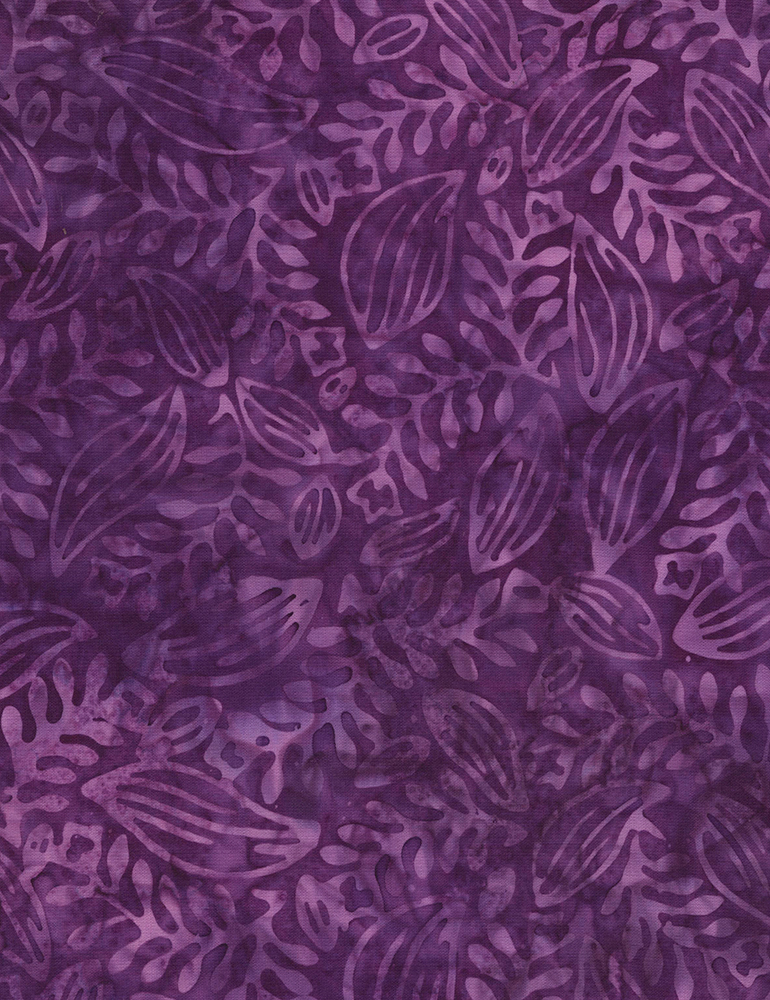# Can you have too many Batiks?

Yeah, that's a pretty silly question! That must be why I am continually bringing more Batiks into the shop!
This new batch of Tonga Batiks is so colorful - you just might need some of each!

 table div table+table+table+table+table+table+table+table+table+table+table+table+table+table+table div table{width:100%;padding:0}table div table+table+table+table+table+table+table+table+table+table+table+table+table+table+table div table img{width:96.23%;padding:0;float:none}table div table+table+table+table+table+table+table+table+table+table+table+table+table+table+table div table td{width:100%;padding:0 1.88% 18px}/* styles */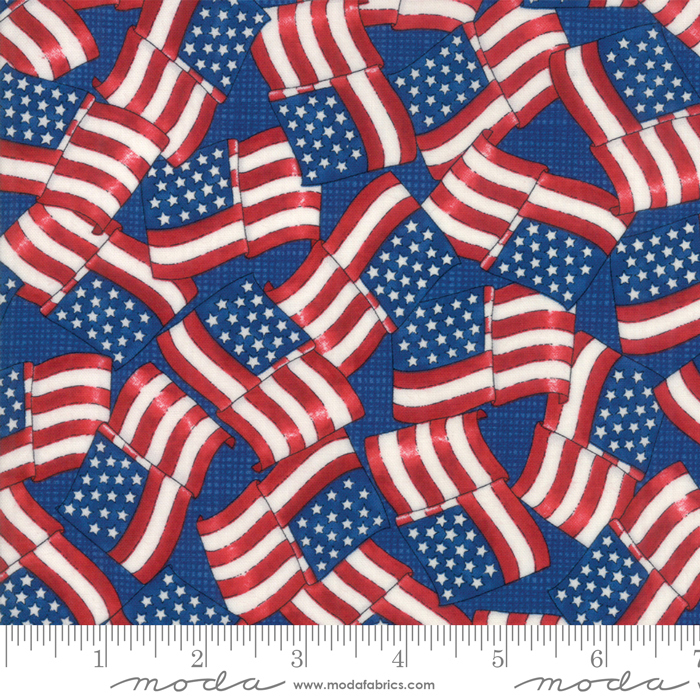# Land That I Love

Great Americana/Patriotic group from Deb Strain!
Several patterns that were designed with this group in mind and we are finally getting ready to cut the Crusin' kits - had to wait for a couple of fabrics to arrive.

 table div table+table+table+table+table+table+table+table+table+table+table+table+table+table+table+table+table+table div table{width:100%;padding:0}table div table+table+table+table+table+table+table+table+table+table+table+table+table+table+table+table+table+table div table img{width:96.23%;padding:0;float:none}table div table+table+table+table+table+table+table+table+table+table+table+table+table+table+table+table+table+table div table td{width:100%;padding:0 1.88% 18px}/* styles */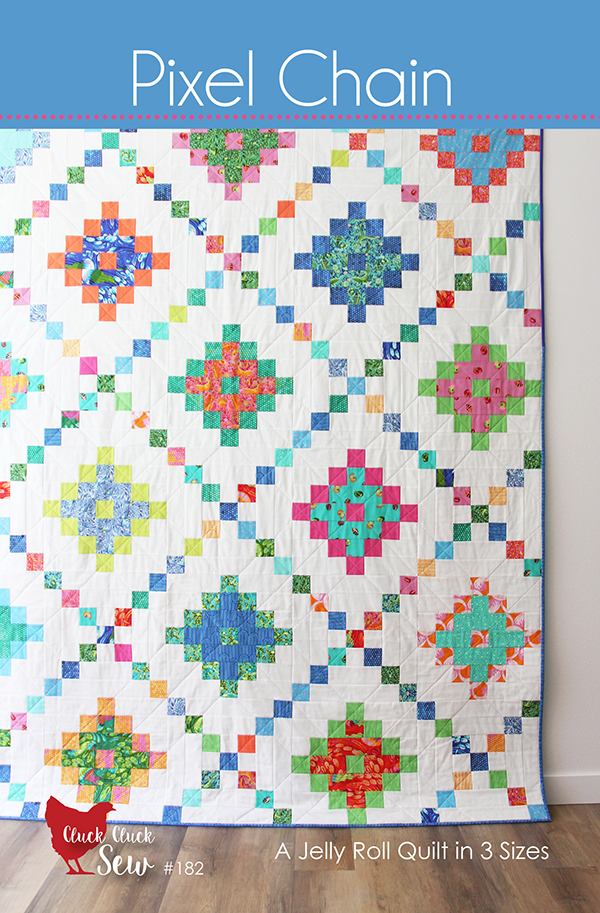# New Books and Patterns!

Great new patterns this week -2 that use precuts, a bag pattern that is perfect for using cork or a textured fabric and a book full of projects for simple wool-applique, embroidery, and quilting techniques - be sure to check them all out!

 table div table+table+table+table+table+table+table+table+table+table+table+table+table+table+table+table+table+table+table+table+table div table{width:100%;padding:0}table div table+table+table+table+table+table+table+table+table+table+table+table+table+table+table+table+table+table+table+table+table div table img{width:96.23%;padding:0;float:none}table div table+table+table+table+table+table+table+table+table+table+table+table+table+table+table+table+table+table+table+table+table div table td{width:100%;padding:0 1.88% 18px}/* styles */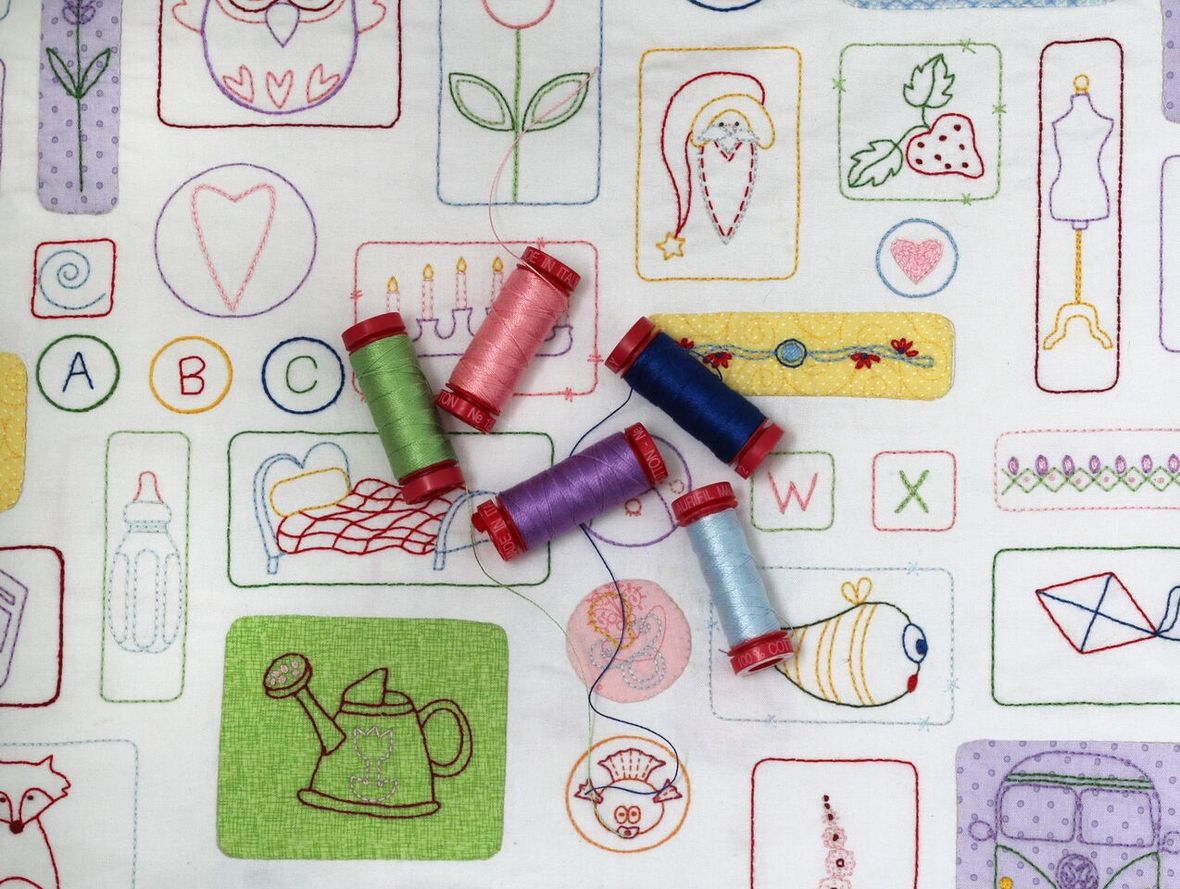# Stitch Therapy 365

It's not too late!
More panels and thread kits have arrived AND...
your 365 days start when you get signed up, so no playing catch up, just a little stitching every day.

 table div table+table+table+table+table+table+table+table+table+table+table+table+table+table+table+table+table+table+table+table+table+table+table div table{width:100%;padding:0}table div table+table+table+table+table+table+table+table+table+table+table+table+table+table+table+table+table+table+table+table+table+table+table div table img{width:96.23%;padding:0;float:none}table div table+table+table+table+table+table+table+table+table+table+table+table+table+table+table+table+table+table+table+table+table+table+table div table td{width:100%;padding:0 1.88% 18px}/* styles */# Next PMS Night is Friday, March 15

6pm to midnight
Treat yourself to a night of food, fabric and friends!

 table div table+table+table+table+table+table+table+table+table+table+table+table+table+table+table+table+table+table+table+table+table+table+table+table+table+table div table{width:100%;padding:0}table div table+table+table+table+table+table+table+table+table+table+table+table+table+table+table+table+table+table+table+table+table+table+table+table+table+table div table img{width:96.23%;padding:0;float:none}table div table+table+table+table+table+table+table+table+table+table+table+table+table+table+table+table+table+table+table+table+table+table+table+table+table+table div table td{width:100%;padding:0 1.88% 18px}/* styles */# Casual Sew 10am to 4pm

Friday, February 15, 22

# Upcoming Classes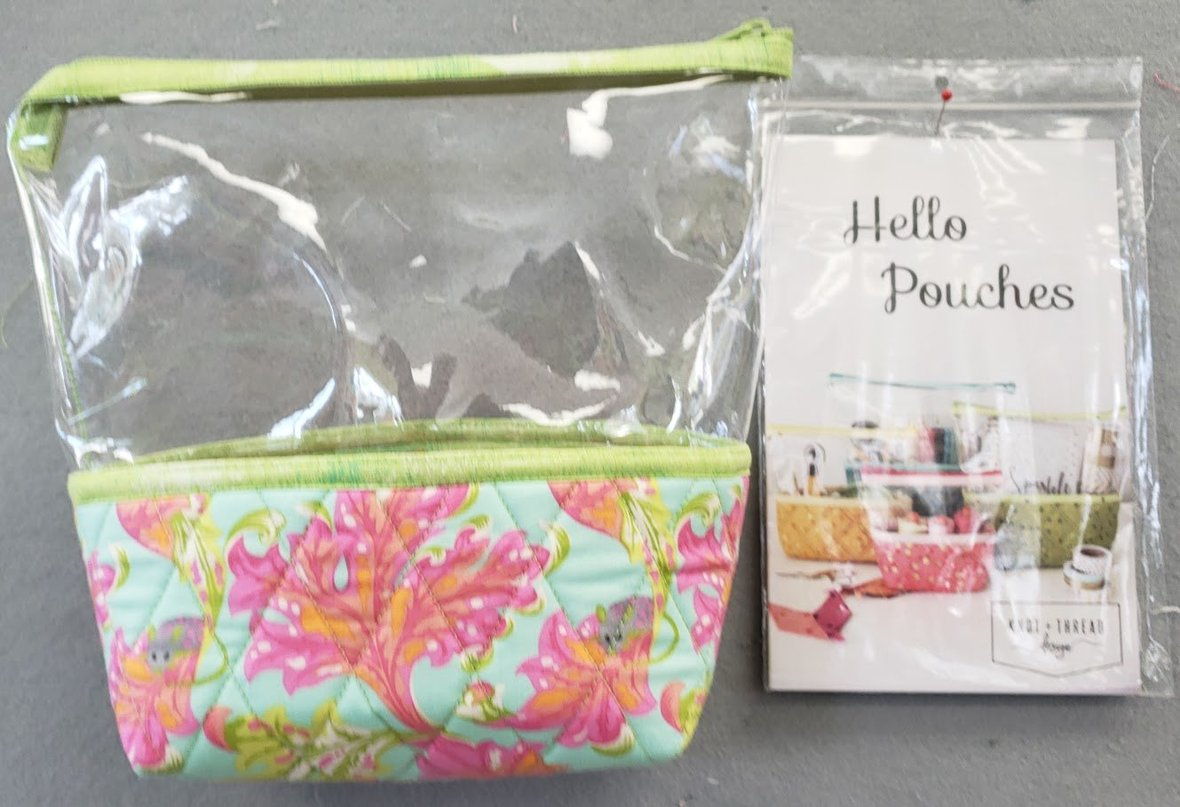# Hello Pouches

It's your new favorite bag!
class is Saturday, February 16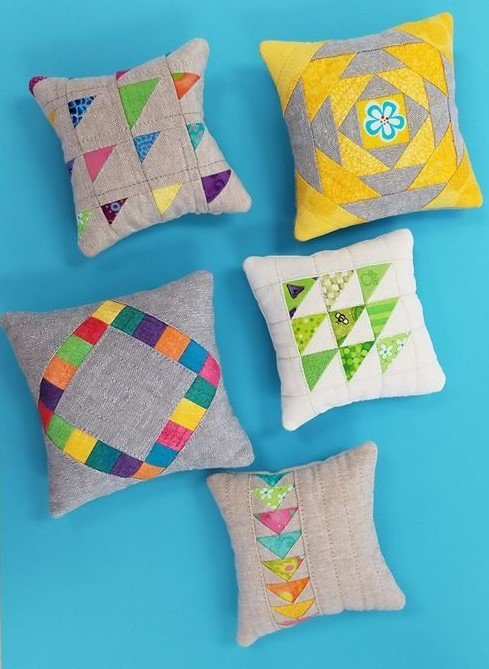# Plenty of Pincushions

Such a fun class - learn how to sew these tiny pieces and finish one in class!
class is Sunday, February 24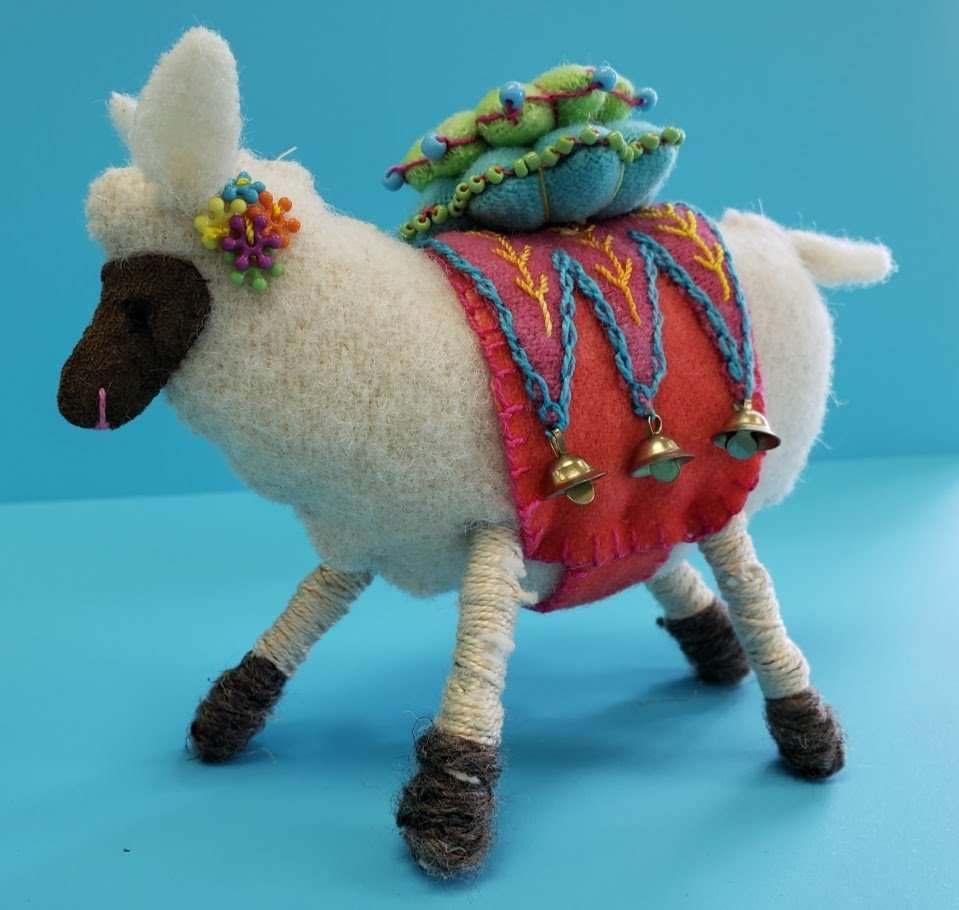# Ewenice

Make this adorable sheep pincushion! Suzi will teach you how to make her special with stitches and embellishments!
class is Tuesday, February 26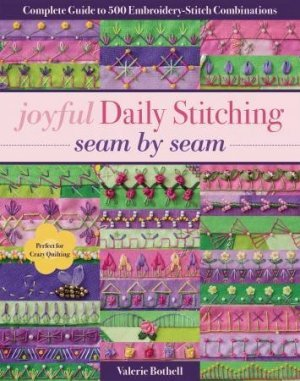# Day Stitchers

Meets Wednesday, February 27
join anytime!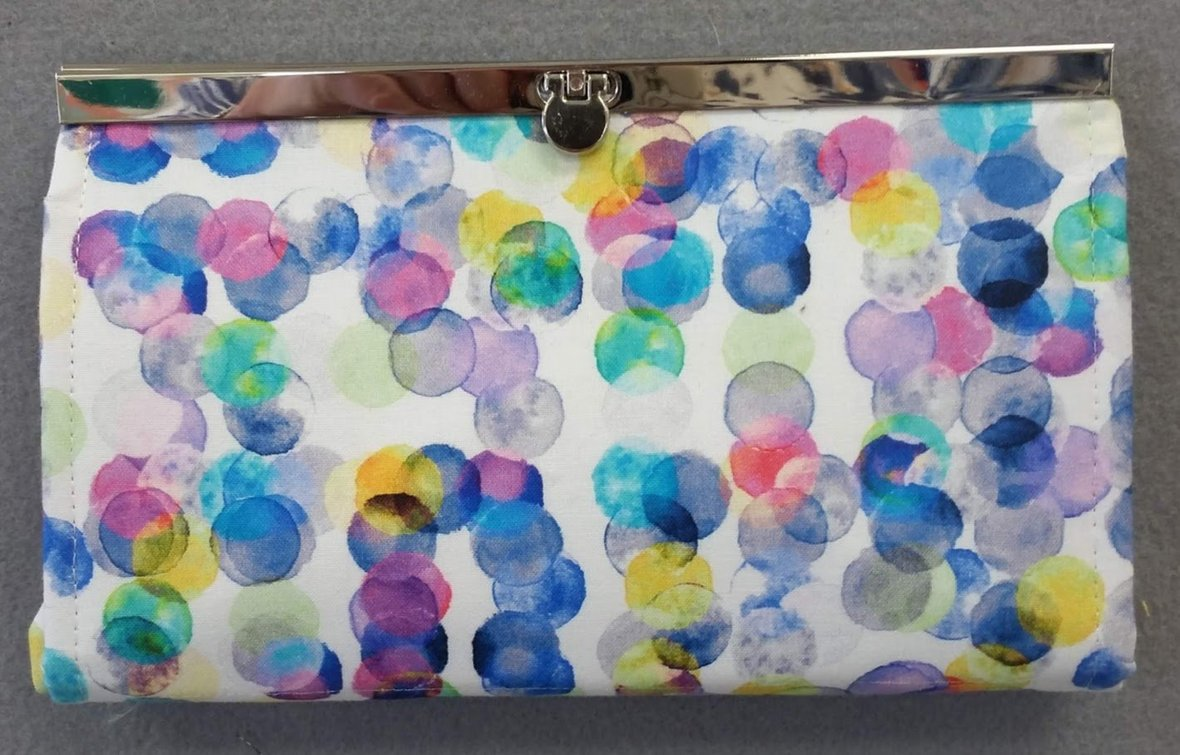# Perfect Wallet

Make one for yourself or a gift, so many great features to this wallet!
class is Thursday, February 28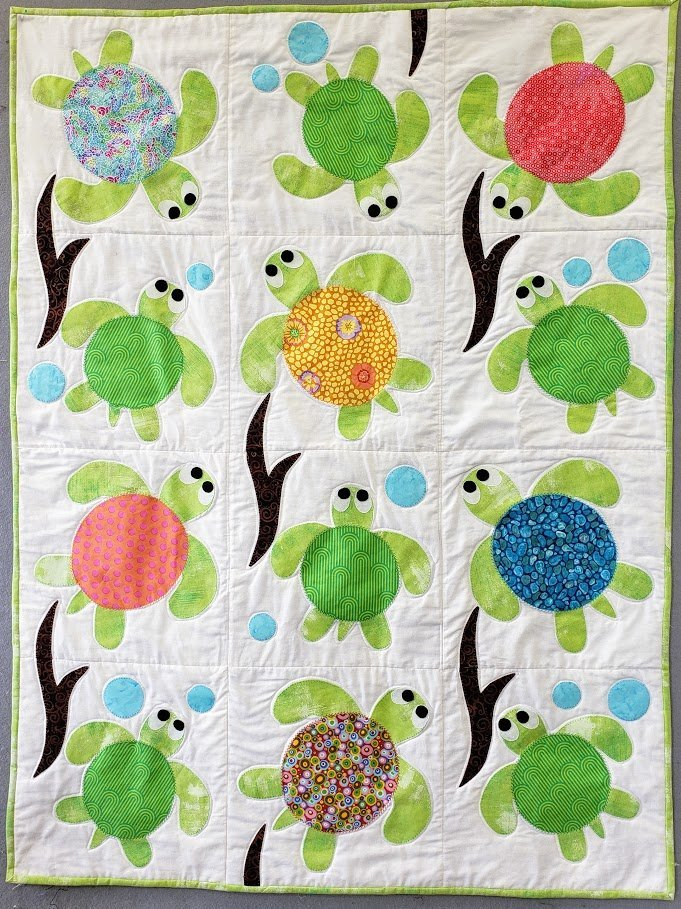# Sea Turtle Friends

An adorable quilt to learn machine applique!
Class is Saturday, March 2

# And there's more!

 table div table+table+table+table+table+table+table+table+table+table+table+table+table+table+table+table+table+table+table+table+table+table+table+table+table+table+table+table+table+table+table+table+table+table+table+table+table+table+table div table{width:100%;padding:0}table div table+table+table+table+table+table+table+table+table+table+table+table+table+table+table+table+table+table+table+table+table+table+table+table+table+table+table+table+table+table+table+table+table+table+table+table+table+table+table div table img{width:96.23%;padding:0;float:none}table div table+table+table+table+table+table+table+table+table+table+table+table+table+table+table+table+table+table+table+table+table+table+table+table+table+table+table+table+table+table+table+table+table+table+table+table+table+table+table div table td{width:100%;padding:0 1.88% 18px}/* styles */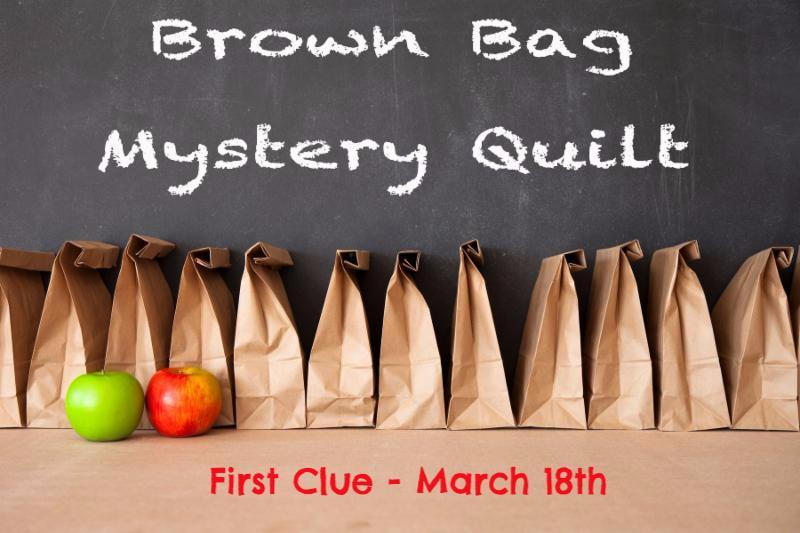# 2019 Brown Bag Mystery is ready!

If you joined us last year, you know what fun it is!
It's a true mystery - you don't know what the pattern is and you don't know what all the fabrics are!
The bow on the bag is your only "clue" - if you like that fabric, you will like your fabrics.

And, yes, you can play even if you live far away!

# 2019 Brown Bag Mystery is ready!

If you joined us last year, you know what fun it is!
It's a true mystery - you don't know what the pattern is and you don't know what all the fabrics are!
The bow on the bag is your only "clue" - if you like that fabric, you will like your fabrics.

And, yes, you can play even if you live far away!

## ps, the Creative Grids tool that the designer recommended is in stock now! Click on the link below.

 table div table+table+table+table+table+table+table+table+table+table+table+table+table+table+table+table+table+table+table+table+table+table+table+table+table+table+table+table+table+table+table+table+table+table+table+table+table+table+table+table+table+table+table+table+table+table div table{width:100%;padding:0}table div table+table+table+table+table+table+table+table+table+table+table+table+table+table+table+table+table+table+table+table+table+table+table+table+table+table+table+table+table+table+table+table+table+table+table+table+table+table+table+table+table+table+table+table+table+table div table img{width:96.23%;padding:0;float:none}table div table+table+table+table+table+table+table+table+table+table+table+table+table+table+table+table+table+table+table+table+table+table+table+table+table+table+table+table+table+table+table+table+table+table+table+table+table+table+table+table+table+table+table+table+table+table div table td{width:100%;padding:0 1.88% 18px}/* styles */# Open 7 days a week for your convenience!

Monday through Saturday, 10am to 5pm
Sunday 12pm to 4pm

See you soon!

 table div table+table+table+table+table+table+table+table+table+table+table+table+table+table+table+table+table+table+table+table+table+table+table+table+table+table+table+table+table+table+table+table+table+table+table+table+table+table+table+table+table+table+table+table+table+table+table+table div table{width:100%;padding:0}table div table+table+table+table+table+table+table+table+table+table+table+table+table+table+table+table+table+table+table+table+table+table+table+table+table+table+table+table+table+table+table+table+table+table+table+table+table+table+table+table+table+table+table+table+table+table+table+table div table img{width:96.23%;padding:0;float:none}table div table+table+table+table+table+table+table+table+table+table+table+table+table+table+table+table+table+table+table+table+table+table+table+table+table+table+table+table+table+table+table+table+table+table+table+table+table+table+table+table+table+table+table+table+table+table+table+table div table td{width:100%;padding:0 1.88% 18px}/* styles *//* styles */ My handsome Freddie!
 table.module-49{width:63.77%;padding:0}table div table+table+table+table+table+table+table+table+table+table+table+table+table+table+table+table+table+table+table+table+table+table+table+table+table+table+table+table+table+table+table+table+table+table+table+table+table+table+table+table+table+table+table+table+table+table+table+table+table+table div table{width:63.77%;float:none;margin-left:auto;margin-right:auto;padding:0}table div table+table+table+table+table+table+table+table+table+table+table+table+table+table+table+table+table+table+table+table+table+table+table+table+table+table+table+table+table+table+table+table+table+table+table+table+table+table+table+table+table+table+table+table+table+table+table+table+table+table div table a{border:0 none;text-decoration:none}table div table+table+table+table+table+table+table+table+table+table+table+table+table+table+table+table+table+table+table+table+table+table+table+table+table+table+table+table+table+table+table+table+table+table+table+table+table+table+table+table+table+table+table+table+table+table+table+table+table+table div table img{width:100%!important;border:0 none;text-decoration:none}table div table+table+table+table+table+table+table+table+table+table+table+table+table+table+table+table+table+table+table+table+table+table+table+table+table+table+table+table+table+table+table+table+table+table+table+table+table+table+table+table+table+table+table+table+table+table+table+table+table+table div table td{width:100%;padding:0}/* styles */
 Like   Pin   +1